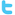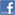Genesis Insoft The name you can TRUSTHome  |  Contact Us |  Careers
C++ Question Bank / FAQs

This is a mock Exam for the C++ programmers. It is created by Genesis InSoft Limited (admin@genesisinsoft.com) and may be freely distributed so long as it is unmodified. Please email us if you have any corrections or comments.

##### Question 1

What does an empty class contain?

1. Default constructor
2. Copy constructor
4. All of the above
##### Question 2

In protected derivation

1. Protected and public members of base class become protected
2. Private, protected and public members of base class become protected
3. Private, protected and public members  of base class become private
4. Protected and public members of base class become private
##### Question 3

What is the output of the program?

#include <iostream.h>

void main()
{
int j = 20, k = 30;
int & m = j;
int * n = &j;
cout << j << " " << k << " " << m << " ++ " ;
m = k;
cout << j << " " << k << " " << m << " ++ ";
n = &k; // n now points to k
cout << j << " " << k << " " << m << " " << *n << endl;
}

1. 20 30 20 ++ 20 30 30 ++ 30 30 30 30
2. 20 30 20 ++ 30 30 30 ++ 20 30 30 30
3. 20 30 20 ++ 20 30 30 ++ 20 30 30 30
4. 20 30 20 ++ 30 30 30 ++ 30 30 30 30
##### Question 4

Class istream in iostream.h is defined as

1. Class istream : public ios
2. Class istream : public virtual ios
3. Class istream : public iostream
4. Class istream : public virtual iostream
##### Question 5

Interface contains

1. At least one pure virtual function
2. No pure virtual function
3. All pure virtual functions
4. None of the above
##### Question 6

What is the size of empty class?

1. 0 bytes
2. 2 bytes
3. 1 byte
4. 4 bytes
##### Question 7

The advantage of defining a pure virtual member function in a class is

1. Derived class may implement the pure virtual function
2. Derived class must implement the pure virtual function
3. Derive class is abstract class if it does not implement the pure virtual function
4. Both B and C
##### Question 8

What is the output of the following?

#include

void main()
{
int x, y;
x=(3, 4, 5);
y=3, 4, 5;
cout << endl <<  x <<"  "<< y;
}

1. Compilation Error
2. 3 5
3. 3 3
4. 5 3
##### Question 9

What is the output of the following?

#include <iostream.h>

void main ()
{
{
for(int x=1; x <= 5; x++, x+=5);
}
cout << endl << " value of x = " << x;
}

1. 6
2. 7
3. compilation error
4. 2
##### Question 10

What is the output of the following?

#include <iostream.h>

void main ()
{
cout << (cout<<" Hello ") << " world ";
}

1. No output is displayed
3. Hello world
4. compilation error
##### Question 11

The class fstreambuf serves as base class for

1. ifstream, ofstream, fstream
2. ifstream, ofstream
3. ostream
4. ifstream
##### Question 12

The scope resolution operator permits

1. Access to an identifier in the global scope that has been hidden by another identifier with the same name in the local scope
4. Access to an identifier in the local scope and global scope
##### Question 13

The declaration
void func_name( )
accepts

1. Any no of arguments
2. Only one argument
3. No Arguments
4. None of the above
##### Question 14

The technique of allocating memory during runtime on demand is known as

1. Dynamic binding
2. Dynamic memory allocation
3. Late binding
4. Template
##### Question 15

What is the output of the following?

#include

void main()
{
enum col {red, blue, yellow};
col c = blue << 1;
cout << c;
} <
1. 1
2. 2
3. 3
4. 4
##### Question 16

The advantage of defining a pure virtual member function in a class is

1. Derived class may implement the pure virtual function
2. Derived class must implement the pure virtual function
3. Derive class is abstract class if it does not implement the pure virtual function
4. Both B and C
##### Question 17

Input and output operators are known as

1. extraction and insertion
2. get from and put to
3. Both A and B
4. None of the above
##### Question 18

A file can be tied to your program by defining an instance of

1. fstream
2. ifstream
3. ofstream
4. All of the above
##### Question 19

Which of the following is not true about constructor

2. constructor return type is int
3. constructor has the same name as the class in which it is defined
4. constructor are used for initializing data members
##### Question 20

What is the output of the program?

#include <iostream.h>
char *buf1 = "Genesis", *buf2 = "InSoft";

void main()
{
const char *p = buf1;
p = buf2;
*p = 'g';
cout << *p;
}

What is the output of the program?

1. g
2. genesis
3. No ouput is displayed
4. l-value specifies constant object